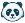# set total amount of points for Quiz instead of each individual question.

I would like to be able to set the overall amount of points a quiz is worth instead of having to assign points to each individual question. I would like to do this because I would like to make every single question on the quiz worth the same and add up to 100. Sometimes this becomes difficult because depending on the number of questions I cannot assign the right amount of points so that the quiz is worth 100 points.

1 CommentCommunity Team
Status changed to: Archived

tl;dr In New Quizzes, you can do this by assigning the points possible on the assignment details page to 100. Then assign each of the quiz question points the same value (could just leave each the default, which is 1 point). When submissions are graded a percentage is made of students' correct answers out of the sum of the individual question points and is applied to the total points possible for the quiz.

Longer Explanation:

This functionality isn't available using the Classic Quizzes tool. However, it is available using the New Quizzes tool.

In New Quizzes, you can assign points for individual questions and points possible for the quiz.

Points possible set on the assignment details page determines the total points the assessment is worth. Points set per question determine points earned for correct answers. Points students earn for correct answers are totaled and divided by the sum of the possible question points to calculate a percentage score for the quiz. That percentage is then used to calculate the final score based on the total points set on the assignment details page.

For example, if you set the total points on the assignment details page to 100 and create an assessment with 10 questions each worth 1 point, the quiz has a total of 10 points but is worth 100 points when graded. If a student answers 9 questions correctly, their quiz score is 90% (9/10). However, their final score in the Gradebook will be 90/100 (90%).

Naomi